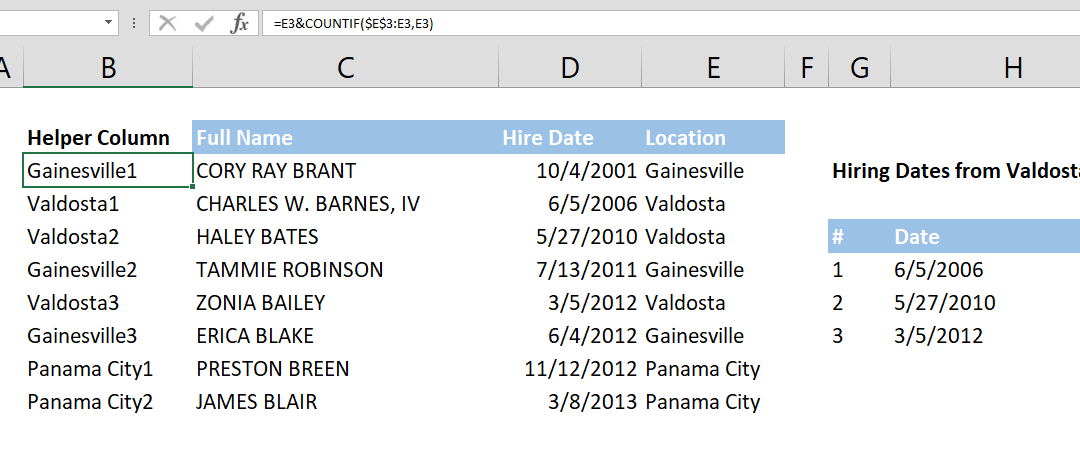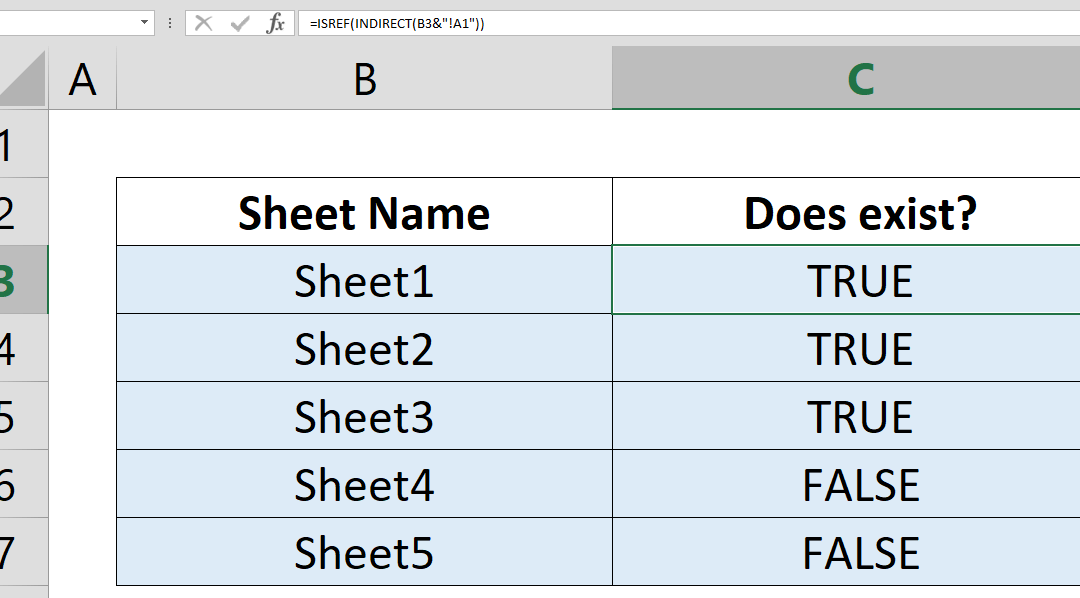## How to SUM values across sheets

To sum range of cells in the same worksheet is one of the basic concepts of Excel. What about summing values across sheets? In this article we will explain how to sum values across sheets. Syntax =SUM(first sheet : second sheet ! reference) Steps Start with =SUM( Type...## How to count values if date is less than by using COUNTIF function

Using the versatile COUNTIF and COUNTIFS functions, see how you can count cells up to a certain date. How to count values if date is less than by using COUNTIF function article shows an essential tip for creating YTD (year-to-date) reports. Syntax =COUNTIFS(date...## How to convert D/M/YYYY date to M/D/YYYY or vice versa

Data formats differ by locations in the world. Once you understand the syntax of dates you can easily convert them from one locale to the other. In this article, we will cover the most standard date conversion in Excel: converting D/M/YYYY to M/D/YYYY. Syntax first...## How to count values if date is greater than by using COUNTIF function

Using the versatile COUNTIF and COUNTIFS functions, see how you can count values if date is greater than from a certain date. An essential tip for creating YTD (year-to-date) reports. Syntax =COUNTIFS(date range, >minimum date) =COUNTIF(date range, >minimum...## How to calculate running count

This article shows how to calculate running count by using COUNTIF and COUNTIFS functions. The running totals help you to track number of value occurrences in your data. Syntax =COUNTIF(expanding range of lookup values, lookup value again) Steps Add a new column to...## How to get nth match with VLOOKUP

This article shows you how to get the nth match with VLOOKUP formula. Unfortunately, Excel doesn’t have a built-in function to find any value beyond the first match. Syntax Unique lookup value: =lookup value & COUNTIF(expanding range of lookup values, lookup...## How to count the number of cells by an equal criteria

Do you have a data table where you need to pick all the highest, lowest, best, worst, or equal to 2? COUNTIF, and its bigger brother COUNTIFS formulas can help sum the cells of your choice! In this article, we’re going to show you how to count the number of...## How to sum filtered values in data tables

Excel’s regular SUM function includes all values in a range even if they are hidden by a filter or the right click Hide feature. This can be quite tedious when trying to create interactive spreadsheets that can utilize such controls. Using he SUBTOTAL function...